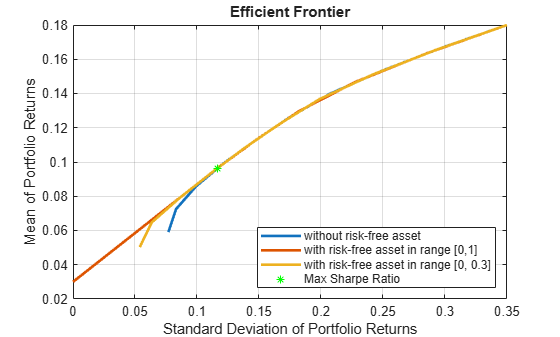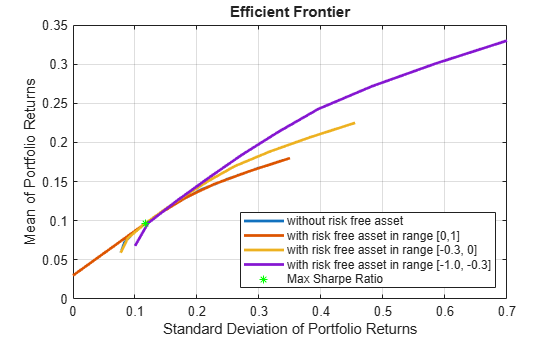# Leverage in Portfolio Optimization with a Risk-Free Asset

This example shows how to use the `setBudget` function for the `Portfolio` class to define the limits on the `sum(AssetWeight_i)` in risky assets.

If the `sum(AssetWeight_i)` is less than `1`, the extra cash is invested in a risk-free asset. If the `sum(AssetWeight_i)` is larger than `1`, meaning that total risky asset investment is larger than initial available cash, the risk-free asset is shorted (borrowed) to fund the extra investment in a risky asset. The cost associated with borrowing a risk-free asset is automatically captured in the mean-variance optimization model for the `Portfolio` class. Therefore, you can use the `setBudget` function directly to control the level of leverage of cash for the portfolio.

### Portfolio Without Leverage

Consider the following example that does not leverage a risk-free asset.

```assetsMean = [ 0.05; 0.1; 0.12; 0.18; ]; assetCovar = [ 0.0064 0.00408 0.00192 0; 0.00408 0.0289 0.0204 0.0119; 0.00192 0.0204 0.0576 0.0336; 0 0.0119 0.0336 0.1225]; riskFreeRate = 0.03; % create a portfolio and define risk-free rate. p = Portfolio('RiskFreeRate', riskFreeRate, 'assetmean', assetsMean, 'assetcovar', assetCovar, 'lowerbound', 0); ```

Create multiple portfolios with different budgets on risky assets to control the limits for investing in a risk-free asset.

```p = setBudget(p, 1, 1); % allow 0% risk free asset allocation, meaning fully invested in risky assets p1 = setBudget(p, 0, 1); % allow 0% to 100% risk free asset allocation p2 = setBudget(p, 0.7, 1); % allow 0% to 30% risk free asset allocation [risk, retn] = estimatePortMoments(p, estimateMaxSharpeRatio(p)); figure; plotFrontier(p); hold on; plotFrontier(p1);hold on; plotFrontier(p2); hold on; plot(risk, retn, 'g*'); hold off; legend('without risk-free asset', ... 'with risk-free asset in range [0,1]', ... 'with risk-free asset in range [0, 0.3]', ... 'Max Sharpe Ratio', 'location','southeast');```In the efficient frontiers in the above figure, the lower-left part of the red efficient frontier line for the portfolio with a risk-free asset is in range `[0,1]` and is actually the capital allocation line (CAL). The slope of this line is the maximum Sharpe ratio of the portfolio, which demonstrates how return is best awarded by taking extra risk. The upper right of the red efficient frontier line is the same as a fully invested portfolio (blue line). Once the portfolio crosses the Sharpe ratio point, the portfolio is fully invested and there is no more cash available to allow high risk-awarded returns following the straight CAL. However, if borrowing of a risk-free asset is allowed, you can effectively use the funds from the borrowing of a risk-free asset to invest in more risky assets, as demonstrated in the "Portfolio with Leverage" section.

### Portfolio with Leverage

To fund investments in risky assets, consider using leverage by borrowing a risk-free asset. The `Portfolio` class enables you to use leverage in asset allocation when a risk-free asset is available in the portfolio.

First, check if the `RiskFreeRate` property for the `Portfolio` object is nonzero.

`p`
```p = Portfolio with properties: BuyCost: [] SellCost: [] RiskFreeRate: 0.0300 AssetMean: [4x1 double] AssetCovar: [4x4 double] TrackingError: [] TrackingPort: [] Turnover: [] BuyTurnover: [] SellTurnover: [] Name: [] NumAssets: 4 AssetList: [] InitPort: [] AInequality: [] bInequality: [] AEquality: [] bEquality: [] LowerBound: [4x1 double] UpperBound: [] LowerBudget: 1 UpperBudget: 1 GroupMatrix: [] LowerGroup: [] UpperGroup: [] GroupA: [] GroupB: [] LowerRatio: [] UpperRatio: [] MinNumAssets: [] MaxNumAssets: [] BoundType: [] ```

In this `Portfolio` object, the lower and upper budgets are both `1`. Limits must be set on the total investment in risky assets. Borrowing a risk-free asset funds the extra investment in risky assets. Use `setBudget` to set the lower and upper bounds to set the limits of the borrowed risk-free assets.

```p = setBudget(p, 1, 1); % allow 0% risk free asset allocation, meaning fully invested in risky assets p3 = setBudget(p, 1, 1.3); % allow 0% risk free asset allocation, and allow borrowing of risk free asset to reach up to 30% leverage p4 = setBudget(p, 1.3, 2); % allow 0% risk free asset allocation, and allow borrowing of risk free asset to have at least 30% leverage and max 100% leverage [risk, retn] = estimatePortMoments(p, estimateMaxSharpeRatio(p)); figure plotFrontier(p); hold on; plotFrontier(p1);hold on; plotFrontier(p3); hold on; plotFrontier(p4); hold on; plot(risk, retn, 'g*'); hold off; legend('without risk free asset', ... 'with risk free asset in range [0,1]', ... 'with risk free asset in range [-0.3, 0]', ... 'with risk free asset in range [-1.0, -0.3]',... 'Max Sharpe Ratio', 'location','southeast');```In this figure, the upper-right parts of both the orange and purple efficient frontiers extend from the CAL (lower-left red line), because of the leverage of a risk-free asset. The same levels of risk-awarded returns are obtained. Once the portfolio exhausts the maximum allowed leverage, the efficient frontier starts to fall below the CAL again, resulting in portfolios with lower Sharpe ratios.